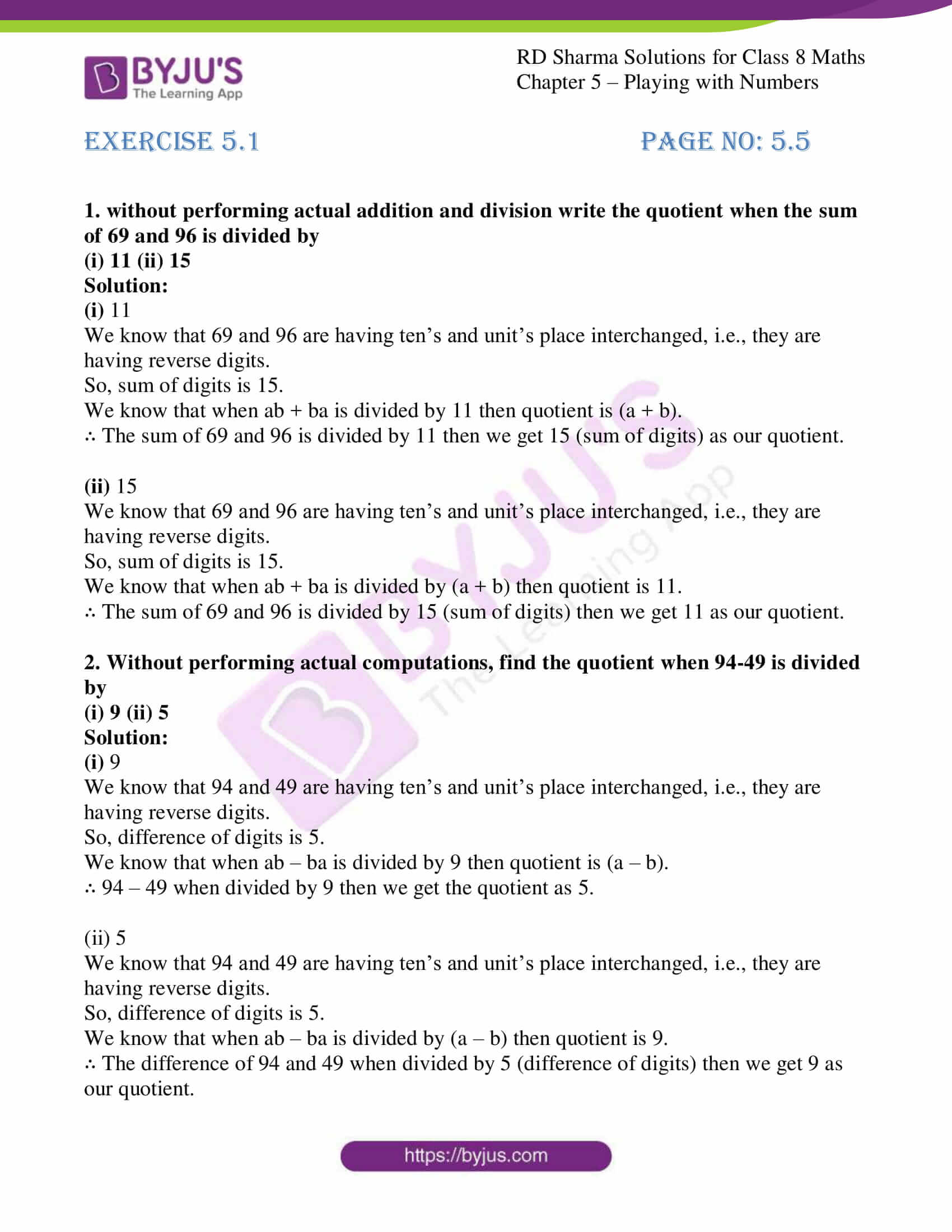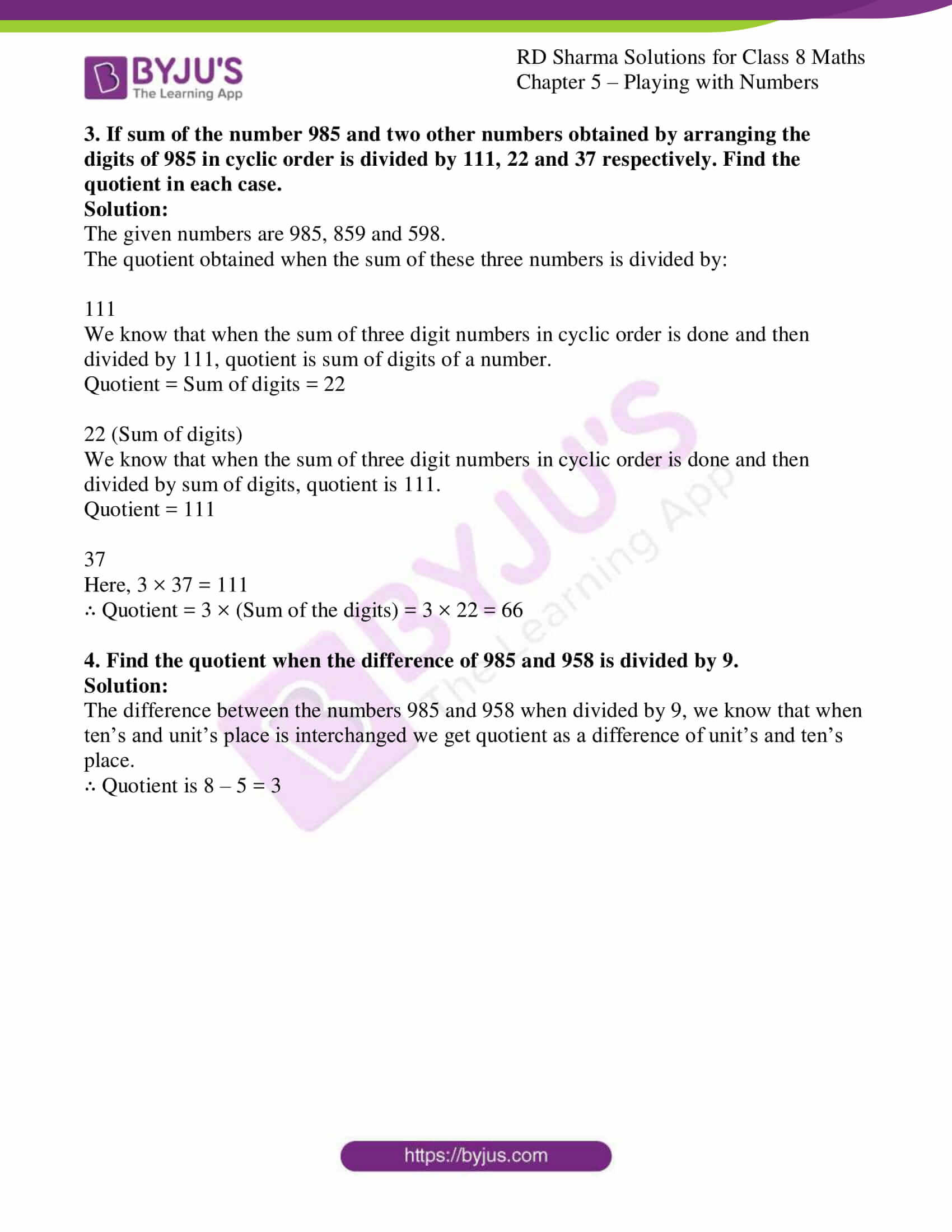# RD Sharma Solutions for Class 8 Maths Chapter 5 - Playing with Numbers Exercise 5.1

In Exercise 5.1 of RD Sharma Class 8 Maths Chapter 5 Playing with Numbers, we shall discuss problems based on the generalized form of numbers, interchanging the digits of a number. Students can refer and download RD Sharma Solutions for Class 8 Maths from the links provided. Our expert tutors at BYJU’S have solved the RD Sharma Class 8 Solutions to ensure that the students have in-depth knowledge of the basic concepts by practising the solutions.

## Download the pdf of RD Sharma Solutions For Class 8 Maths Chapter 5 Playing with Numbers Exercise 5.1### Access answers to Maths RD Sharma Solutions For Class 8 Exercise 5.1 Chapter 5 – Playing with Numbers

1. without performing actual addition and division write the quotient when the sum of 69 and 96 is divided by
(i) 11 (ii) 15

Solution:

(i) 11

We know that 69 and 96 are having ten’s and unit’s place interchanged, i.e., they are having reverse digits.

So, sum of digits is 15.

We know that when ab + ba is divided by 11 then quotient is (a + b).

∴ The sum of 69 and 96 is divided by 11 then we get 15 (sum of digits) as our quotient.

(ii) 15

We know that 69 and 96 are having ten’s and unit’s place interchanged, i.e., they are having reverse digits.

So, sum of digits is 15.

We know that when ab + ba is divided by (a + b) then quotient is 11.

∴ The sum of 69 and 96 is divided by 15 (sum of digits) then we get 11 as our quotient.

2. Without performing actual computations, find the quotient when 94-49 is divided by
(i) 9 (ii) 5

Solution:

(i) 9

We know that 94 and 49 are having ten’s and unit’s place interchanged, i.e., they are having reverse digits.

So, difference of digits is 5.

We know that when ab – ba is divided by 9 then quotient is (a – b).

∴ 94 – 49 when divided by 9 then we get the quotient as 5.

(ii) 5

We know that 94 and 49 are having ten’s and unit’s place interchanged, i.e., they are having reverse digits.

So, difference of digits is 5.

We know that when ab – ba is divided by (a – b) then quotient is 9.

∴ The difference of 94 and 49 when divided by 5 (difference of digits) then we get 9 as our quotient.

3. If sum of the number 985 and two other numbers obtained by arranging the digits of 985 in cyclic order is divided by 111, 22 and 37 respectively. Find the quotient in each case.

Solution:

The given numbers are 985, 859 and 598.

The quotient obtained when the sum of these three numbers is divided by:

111

We know that when the sum of three digit numbers in cyclic order is done and then divided by 111, quotient is sum of digits of a number.

Quotient = Sum of digits = 22

22 (Sum of digits)

We know that when the sum of three digit numbers in cyclic order is done and then divided by sum of digits, quotient is 111.

Quotient = 111

37

Here, 3 × 37 = 111

∴ Quotient = 3 × (Sum of the digits) = 3 × 22 = 66

4. Find the quotient when the difference of 985 and 958 is divided by 9.

Solution:

The difference between the numbers 985 and 958 when divided by 9, we know that when ten’s and unit’s place is interchanged we get quotient as a difference of unit’s and ten’s place.

∴ Quotient is 8 – 5 = 3